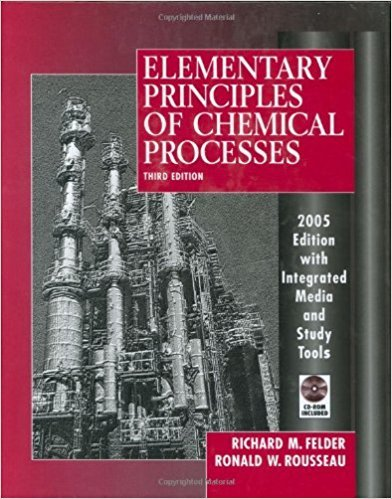×
Get Full Access to Elementary Principles Of Chemical Processes - 3 Edition - Chapter 8 - Problem 8.2
Get Full Access to Elementary Principles Of Chemical Processes - 3 Edition - Chapter 8 - Problem 8.2

×

# The heat capacity at constant pressure of hydrogen cyanideISBN: 9780471687573 143

## Solution for problem 8.2 Chapter 8

Elementary Principles of Chemical Processes | 3rd Edition

• Textbook Solutions
• 2901 Step-by-step solutions solved by professors and subject experts
• Get 24/7 help from StudySoup virtual teaching assistantsElementary Principles of Chemical Processes | 3rd Edition

4 5 1 296 Reviews
17
3
Problem 8.2

The heat capacity at constant pressure of hydrogen cyanide is given by the expression Cp[J/(mol'C)] = 35.3 + 0.0291T(C) (a) Write an expression for the heat capacity at constant volume for HCN, assuming ideal gas behavior. (b) Calculate LiH(1/mol) for the constant-pressure process HCN(v. 25C, 0.80 atm) -,> HCN(v. 100C, 0.80 atm) (c) Calculate LiO(1/mol) for the constant-volume process HCN(v. 25C, 50 m3 /kmol) -> HCN(v, 100C, 50 m3 /kmol) (d) If the process of part (b) were carried out in such a way that the initial and final pressures were each 0.80 atm but the pressure varied during the heating, the value of LiH would still be what you calculated assuming a constant pressure. Why is this so?

Step-by-Step Solution:
Step 1 of 3

Organic Chemistry Equilibrium constant eA + fB  sC + tD ­ K eq[C] [D] / [A] [B] f Thermodynamics Y Z Thermodynamics: how much of ‘Z’ is formed Kinetics: How fast ‘Z’ is formed ∆G⁰ =­RTln K eq logK eq pka (conjugate acid)­ pka (acid) ∆G⁰ = ∆H⁰ – T ∆S⁰ Bond dissociation Energy (BDE) = BDE (bonds broken) – BDE (bonds formed) H­H + CH – C3 CH  CH 2 CH – C3 2 H H Rate = frequency of collision X fraction of proper orientation X fraction with sufficient (How fast) (location) (energy)

Step 2 of 3

Step 3 of 3

##### ISBN: 9780471687573

Unlock Textbook Solution